#### You may also like### Pebbles

Place four pebbles on the sand in the form of a square. Keep adding as few pebbles as necessary to double the area. How many extra pebbles are added each time?### It Figures

Suppose we allow ourselves to use three numbers less than 10 and multiply them together. How many different products can you find? How do you know you've got them all?### Bracelets

Investigate the different shaped bracelets you could make from 18 different spherical beads. How do they compare if you use 24 beads?

# Number Tracks

## Number Tracks

Ben's class were making number tracks and cutting them up.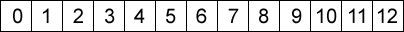... ... ...

First they cut them into twos. Ben started with zero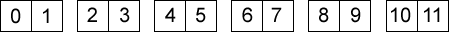... ... ...

but Miles started with one: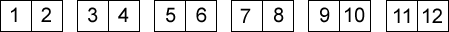... ... ...

Then they both added up the numbers on each piece.

Ben wrote: $0 + 1 = 1$, $2 + 3 = 5$, $4 + 5 =$... ... ...

Miles wrote: $1 + 2 = 3$, $3 + 4 = 7$, $5 + 6 =$ ... ... ...

What patterns could they see?

Alice cut her number track into $3$s and added up the numbers on each one.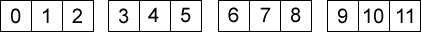... ... ...

Winston made a longer number track which he cut into $5$s and he added up the numbers on each one.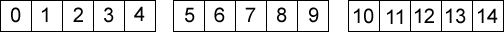... ... ...

What could they discover about the sum of the numbers on their strips of number track?

What other patterns can you find?

### Why do this problem?

This problem is a great opportunity for children to explore numbers in a familiar context in a way that is likely to be unfamiliar. This problem will also help to reinforce important number concepts and vocabulary such as odd and even numbers, factors and multiples, sequences and square numbers. It provides a way in which learners can visualise number patterns and sequences.

### Possible approach

You could start with the whole group by cutting up a large size number strip and adding the two resulting numbers together and writing these on the board. Alternatively, you could use the illustrations in the problem itself.

NOTE: These strips are not number lines which are counted from the division between the spaces, but number tracks in which the spaces themselves are numbered.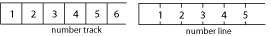Before starting on the actual problem it might be useful to count together in twos, both even and odd numbers, and perhaps in threes and fives as well.

After this learners should work in pairs on the problem with scissors and plenty of number strips. These sheets, which can be printed off and enlarged if necessary, have strips which go from both $0 - 19$ and $1 - 20$. If learners work in pairs they are able to talk through their ideas with their partner. Listening to the pairs talking should help you to assess their understanding of multiples and other sequences.

At the end of the lesson there should be a good discussion on the various number patterns that have been found. How do they think strips cut into sevens would go? What about fours and other even numbers?

The cut strips and resulting added numbers could make an interesting display.

### Key questions

Are you starting with zero or one this time?
Why don't you write down the numbers you have found in a list?
How does your sequence of numbers go?
How do the numbers increase each time?
In what multiplication tables are the numbers you get from adding the $3$ strips and the $5$ strips?
In what way do your list of numbers increase each time?

### Possible extension

Learners could try higher numbers without the support of actually cutting the number tracks. Can they find a pattern for cutting numbers into fours and sixes?

### Possible support

This activity is so accessible that the beginning should not prove too difficult for anyone. Suggest writing down the result of adding the two numbers in order and saying them out loud. Where have they heard these numbers before?